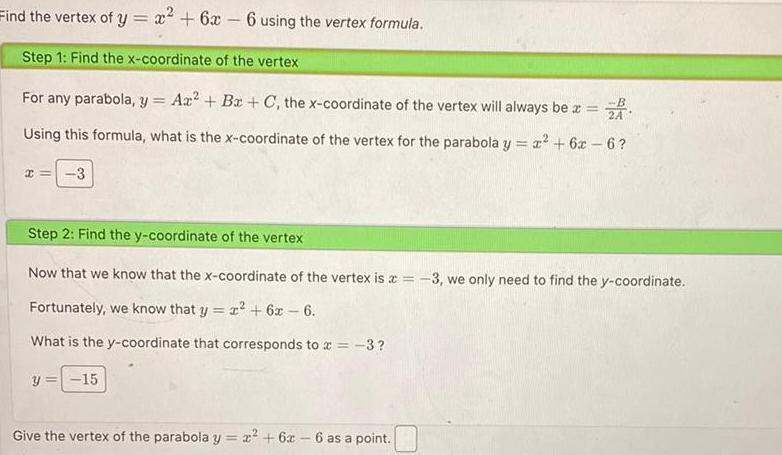Question:

# Find the vertex of y x 6x 6 using the vertex formula Step 1

Last updated: 11/20/2023Find the vertex of y x 6x 6 using the vertex formula Step 1 Find the x coordinate of the vertex For any parabola y Ax Bx C the x coordinate of the vertex will always be x ZA Using this formula what is the x coordinate of the vertex for the parabola y x 6x 6 x 3 Step 2 Find the y coordinate of the vertex Now that we know that the x coordinate of the vertex is x 3 we only need to find the y coordinate Fortunately we know that y x 6x 6 What is the y coordinate that corresponds to x 3 y 15 Give the vertex of the parabola y x 6x 6 as a point